# Matrix.pptx

31 May 2023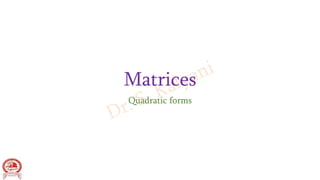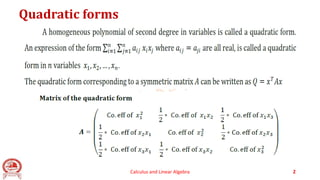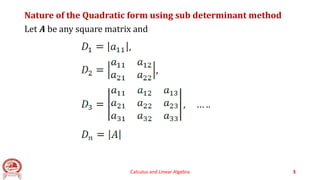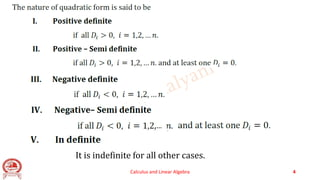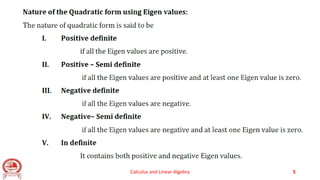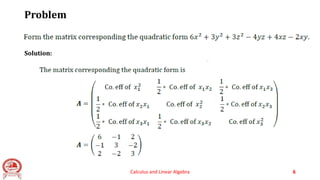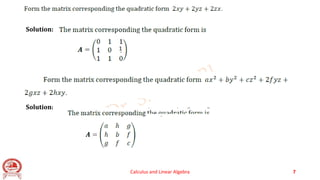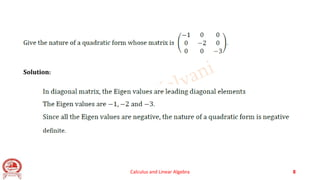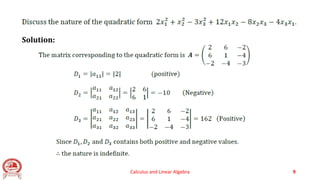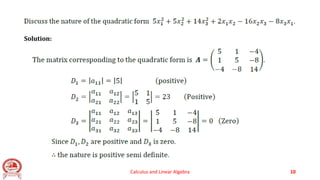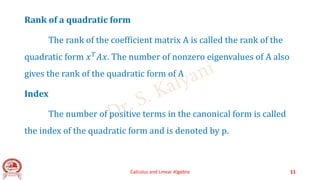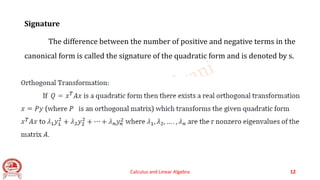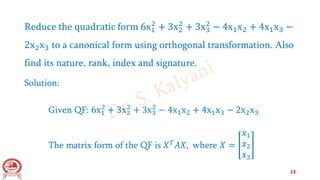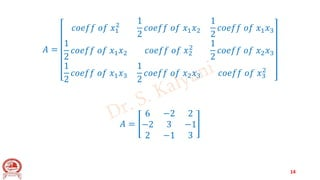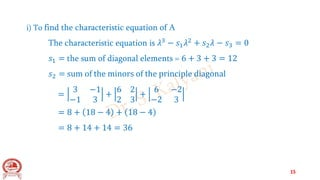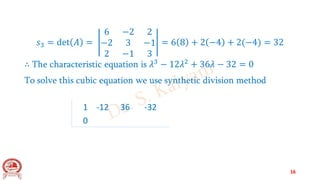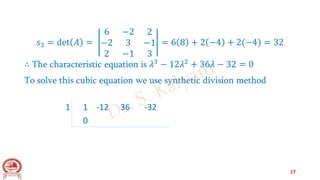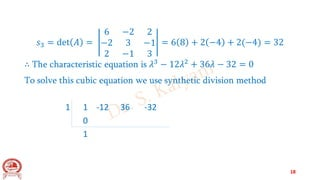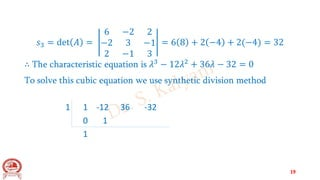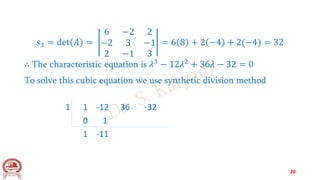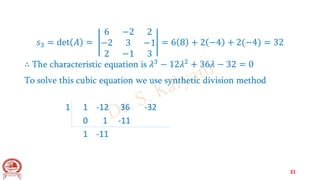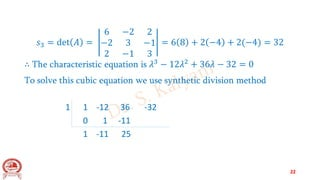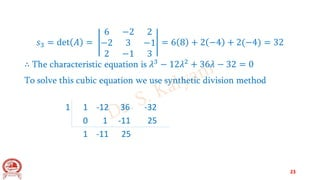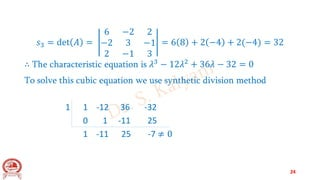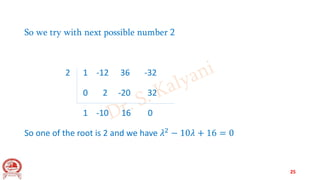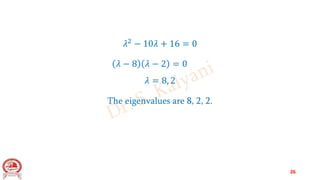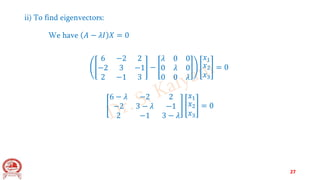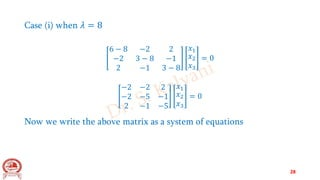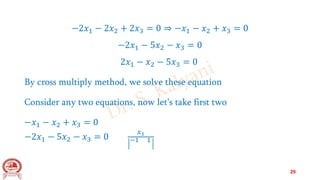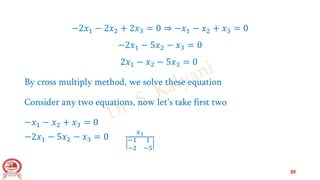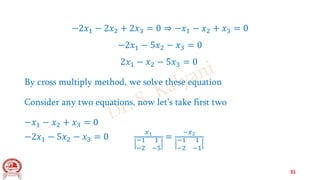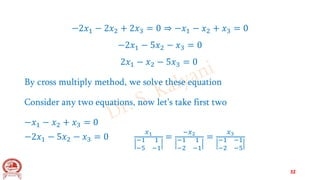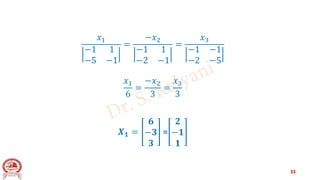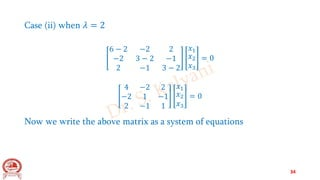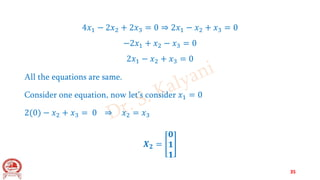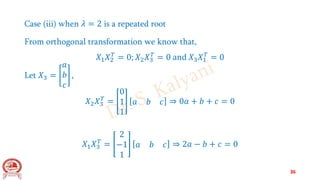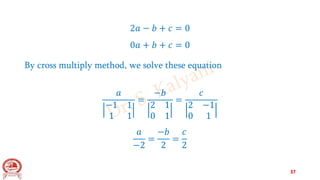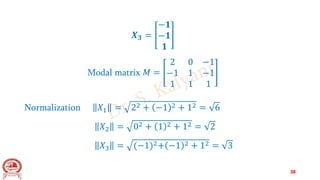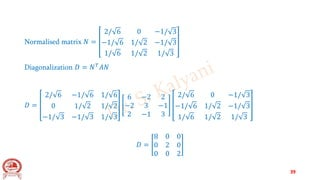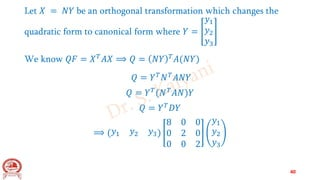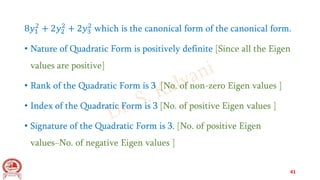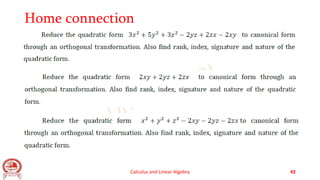1 sur 42

### Matrix.pptx

• 2. Quadratic forms Calculus and Linear Algebra 2
• 3. Nature of the Quadratic form using sub determinant method Let 𝑨 be any square matrix and Calculus and Linear Algebra 3
• 4. Calculus and Linear Algebra 4 It is indefinite for all other cases. ,…
• 5. Calculus and Linear Algebra 5
• 6. Problem Calculus and Linear Algebra 6 Solution:
• 7. Calculus and Linear Algebra 7 Solution: Solution: 1
• 8. Calculus and Linear Algebra 8 Solution:
• 9. Calculus and Linear Algebra 9 Solution:
• 10. Calculus and Linear Algebra 10 Solution:
• 11. Rank of a quadratic form The rank of the coefficient matrix A is called the rank of the quadratic form 𝑥𝑇𝐴𝑥. The number of nonzero eigenvalues of A also gives the rank of the quadratic form of A Index The number of positive terms in the canonical form is called the index of the quadratic form and is denoted by p. Calculus and Linear Algebra 11
• 12. Signature The difference between the number of positive and negative terms in the canonical form is called the signature of the quadratic form and is denoted by s. Calculus and Linear Algebra 12
• 13. Reduce the quadratic form 6x1 2 + 3x2 2 + 3x3 2 − 4x1x2 + 4x1x3 − 2x2x3 to a canonical form using orthogonal transformation. Also find its nature, rank, index and signature. Solution: Given QF: 6x1 2 + 3x2 2 + 3x3 2 − 4x1x2 + 4x1x3 − 2x2x3 The matrix form of the QF is 𝑋𝑇𝐴𝑋, where 𝑋 = 𝑥1 𝑥2 𝑥3 13
• 14. 𝐴 = 𝑐𝑜𝑒𝑓𝑓 𝑜𝑓 𝑥1 2 1 2 𝑐𝑜𝑒𝑓𝑓 𝑜𝑓 𝑥1𝑥2 1 2 𝑐𝑜𝑒𝑓𝑓 𝑜𝑓 𝑥1𝑥3 1 2 𝑐𝑜𝑒𝑓𝑓 𝑜𝑓 𝑥1𝑥2 𝑐𝑜𝑒𝑓𝑓 𝑜𝑓 𝑥2 2 1 2 𝑐𝑜𝑒𝑓𝑓 𝑜𝑓 𝑥2𝑥3 1 2 𝑐𝑜𝑒𝑓𝑓 𝑜𝑓 𝑥1𝑥3 1 2 𝑐𝑜𝑒𝑓𝑓 𝑜𝑓 𝑥2𝑥3 𝑐𝑜𝑒𝑓𝑓 𝑜𝑓 𝑥3 2 𝐴 = 6 −2 2 −2 3 −1 2 −1 3 14
• 15. i) To find the characteristic equation of A The characteristic equation is 𝜆3 − 𝑠1𝜆2 + 𝑠2𝜆 − 𝑠3 = 0 𝑠1 = the sum of diagonal elements = 6 + 3 + 3 = 12 𝑠2 = sum of the minors of the principle diagonal = 3 −1 −1 3 + 6 2 2 3 + 6 −2 −2 3 = 8 + 18 − 4 + 18 − 4 = 8 + 14 + 14 = 36 15
• 16. 𝑠3 = det 𝐴 = 6 −2 2 −2 3 −1 2 −1 3 = 6 8 + 2 −4 + 2(−4) = 32 ∴ The characteristic equation is 𝜆3 − 12𝜆2 + 36𝜆 − 32 = 0 To solve this cubic equation we use synthetic division method 1 -12 36 -32 0 16
• 17. 𝑠3 = det 𝐴 = 6 −2 2 −2 3 −1 2 −1 3 = 6 8 + 2 −4 + 2(−4) = 32 ∴ The characteristic equation is 𝜆3 − 12𝜆2 + 36𝜆 − 32 = 0 To solve this cubic equation we use synthetic division method 1 1 -12 36 -32 0 17
• 18. 𝑠3 = det 𝐴 = 6 −2 2 −2 3 −1 2 −1 3 = 6 8 + 2 −4 + 2(−4) = 32 ∴ The characteristic equation is 𝜆3 − 12𝜆2 + 36𝜆 − 32 = 0 To solve this cubic equation we use synthetic division method 1 1 -12 36 -32 0 1 18
• 19. 𝑠3 = det 𝐴 = 6 −2 2 −2 3 −1 2 −1 3 = 6 8 + 2 −4 + 2(−4) = 32 ∴ The characteristic equation is 𝜆3 − 12𝜆2 + 36𝜆 − 32 = 0 To solve this cubic equation we use synthetic division method 1 1 -12 36 -32 0 1 1 19
• 20. 𝑠3 = det 𝐴 = 6 −2 2 −2 3 −1 2 −1 3 = 6 8 + 2 −4 + 2(−4) = 32 ∴ The characteristic equation is 𝜆3 − 12𝜆2 + 36𝜆 − 32 = 0 To solve this cubic equation we use synthetic division method 1 1 -12 36 -32 0 1 1 -11 20
• 21. 𝑠3 = det 𝐴 = 6 −2 2 −2 3 −1 2 −1 3 = 6 8 + 2 −4 + 2(−4) = 32 ∴ The characteristic equation is 𝜆3 − 12𝜆2 + 36𝜆 − 32 = 0 To solve this cubic equation we use synthetic division method 1 1 -12 36 -32 0 1 -11 1 -11 21
• 22. 𝑠3 = det 𝐴 = 6 −2 2 −2 3 −1 2 −1 3 = 6 8 + 2 −4 + 2(−4) = 32 ∴ The characteristic equation is 𝜆3 − 12𝜆2 + 36𝜆 − 32 = 0 To solve this cubic equation we use synthetic division method 1 1 -12 36 -32 0 1 -11 1 -11 25 22
• 23. 𝑠3 = det 𝐴 = 6 −2 2 −2 3 −1 2 −1 3 = 6 8 + 2 −4 + 2(−4) = 32 ∴ The characteristic equation is 𝜆3 − 12𝜆2 + 36𝜆 − 32 = 0 To solve this cubic equation we use synthetic division method 1 1 -12 36 -32 0 1 -11 25 1 -11 25 23
• 24. 𝑠3 = det 𝐴 = 6 −2 2 −2 3 −1 2 −1 3 = 6 8 + 2 −4 + 2(−4) = 32 ∴ The characteristic equation is 𝜆3 − 12𝜆2 + 36𝜆 − 32 = 0 To solve this cubic equation we use synthetic division method 1 1 -12 36 -32 0 1 -11 25 1 -11 25 -7 ≠ 0 24
• 25. So we try with next possible number 2 2 1 -12 36 -32 0 2 -20 32 1 -10 16 0 So one of the root is 2 and we have 𝜆2 − 10𝜆 + 16 = 0 25
• 26. 𝜆2 − 10𝜆 + 16 = 0 𝜆 − 8 𝜆 − 2 = 0 𝜆 = 8, 2 The eigenvalues are 8, 2, 2. 26
• 27. ii) To find eigenvectors: We have 𝐴 − 𝜆𝐼 𝑋 = 0 6 −2 2 −2 3 −1 2 −1 3 − 𝜆 0 0 0 𝜆 0 0 0 𝜆 𝑥1 𝑥2 𝑥3 = 0 6 − 𝜆 −2 2 −2 3 − 𝜆 −1 2 −1 3 − 𝜆 𝑥1 𝑥2 𝑥3 = 0 27
• 28. Case (i) when 𝜆 = 8 6 − 8 −2 2 −2 3 − 8 −1 2 −1 3 − 8 𝑥1 𝑥2 𝑥3 = 0 −2 −2 2 −2 −5 −1 2 −1 −5 𝑥1 𝑥2 𝑥3 = 0 Now we write the above matrix as a system of equations 28
• 29. −2𝑥1 − 2𝑥2 + 2𝑥3 = 0 ⇒ −𝑥1 − 𝑥2 + 𝑥3 = 0 −2𝑥1 − 5𝑥2 − 𝑥3 = 0 2𝑥1 − 𝑥2 − 5𝑥3 = 0 By cross multiply method, we solve these equation Consider any two equations, now let’s take first two −𝑥1 − 𝑥2 + 𝑥3 = 0 −2𝑥1 − 5𝑥2 − 𝑥3 = 0 𝑥1 −1 1 29
• 30. −2𝑥1 − 2𝑥2 + 2𝑥3 = 0 ⇒ −𝑥1 − 𝑥2 + 𝑥3 = 0 −2𝑥1 − 5𝑥2 − 𝑥3 = 0 2𝑥1 − 𝑥2 − 5𝑥3 = 0 By cross multiply method, we solve these equation Consider any two equations, now let’s take first two −𝑥1 − 𝑥2 + 𝑥3 = 0 −2𝑥1 − 5𝑥2 − 𝑥3 = 0 𝑥1 −1 1 −2 −5 30
• 31. −2𝑥1 − 2𝑥2 + 2𝑥3 = 0 ⇒ −𝑥1 − 𝑥2 + 𝑥3 = 0 −2𝑥1 − 5𝑥2 − 𝑥3 = 0 2𝑥1 − 𝑥2 − 5𝑥3 = 0 By cross multiply method, we solve these equation Consider any two equations, now let’s take first two −𝑥1 − 𝑥2 + 𝑥3 = 0 −2𝑥1 − 5𝑥2 − 𝑥3 = 0 𝑥1 −1 1 −2 −5 = −𝑥2 −1 1 −2 −1 31
• 32. −2𝑥1 − 2𝑥2 + 2𝑥3 = 0 ⇒ −𝑥1 − 𝑥2 + 𝑥3 = 0 −2𝑥1 − 5𝑥2 − 𝑥3 = 0 2𝑥1 − 𝑥2 − 5𝑥3 = 0 By cross multiply method, we solve these equation Consider any two equations, now let’s take first two −𝑥1 − 𝑥2 + 𝑥3 = 0 −2𝑥1 − 5𝑥2 − 𝑥3 = 0 𝑥1 −1 1 −5 −1 = −𝑥2 −1 1 −2 −1 = 𝑥3 −1 −1 −2 −5 32
• 33. 𝑥1 −1 1 −5 −1 = −𝑥2 −1 1 −2 −1 = 𝑥3 −1 −1 −2 −5 𝑥1 6 = −𝑥2 3 = 𝑥3 3 𝑿𝟏 = 𝟔 −𝟑 𝟑 = 𝟐 −𝟏 𝟏 33
• 34. Case (ii) when 𝜆 = 2 6 − 2 −2 2 −2 3 − 2 −1 2 −1 3 − 2 𝑥1 𝑥2 𝑥3 = 0 4 −2 2 −2 1 −1 2 −1 1 𝑥1 𝑥2 𝑥3 = 0 Now we write the above matrix as a system of equations 34
• 35. 4𝑥1 − 2𝑥2 + 2𝑥3 = 0 ⇒ 2𝑥1 − 𝑥2 + 𝑥3 = 0 −2𝑥1 + 𝑥2 − 𝑥3 = 0 2𝑥1 − 𝑥2 + 𝑥3 = 0 All the equations are same. Consider one equation, now let’s consider 𝑥1 = 0 2(0) − 𝑥2 + 𝑥3 = 0 ⇒ 𝑥2 = 𝑥3 𝑿𝟐 = 𝟎 𝟏 𝟏 35
• 36. Case (iii) when 𝜆 = 2 is a repeated root From orthogonal transformation we know that, 𝑋1𝑋2 𝑇 = 0; 𝑋2𝑋3 𝑇 = 0 and 𝑋3𝑋1 𝑇 = 0 Let 𝑋3 = 𝑎 𝑏 𝑐 , 𝑋2𝑋3 𝑇 = 0 1 1 𝑎 𝑏 𝑐 ⇒ 0𝑎 + 𝑏 + 𝑐 = 0 𝑋1𝑋3 𝑇 = 2 −1 1 𝑎 𝑏 𝑐 ⇒ 2𝑎 − 𝑏 + 𝑐 = 0 36
• 37. 2𝑎 − 𝑏 + 𝑐 = 0 0𝑎 + 𝑏 + 𝑐 = 0 By cross multiply method, we solve these equation 𝑎 −1 1 1 1 = −𝑏 2 1 0 1 = 𝑐 2 −1 0 1 𝑎 −2 = −𝑏 2 = 𝑐 2 37
• 38. 𝑿𝟑 = −𝟏 −𝟏 𝟏 Modal matrix 𝑀 = 2 0 −1 −1 1 −1 1 1 1 Normalization 𝑋1 = 22 + −1 2 + 12 = 6 𝑋2 = 02 + 1 2 + 12 = 2 𝑋3 = (−1)2+ −1 2 + 12 = 3 38
• 39. Normalised matrix 𝑁 = 2/ 6 0 −1/ 3 −1/ 6 1/ 2 −1/ 3 1/ 6 1/ 2 1/ 3 Diagonalization 𝐷 = 𝑁𝑇𝐴𝑁 𝐷 = 2/ 6 −1/ 6 1/ 6 0 1/ 2 1/ 2 −1/ 3 −1/ 3 1/ 3 6 −2 2 −2 3 −1 2 −1 3 2/ 6 0 −1/ 3 −1/ 6 1/ 2 −1/ 3 1/ 6 1/ 2 1/ 3 𝐷 = 8 0 0 0 2 0 0 0 2 39
• 40. Let 𝑋 = 𝑁𝑌 be an orthogonal transformation which changes the quadratic form to canonical form where 𝑌 = 𝑦1 𝑦2 𝑦3 We know 𝑄𝐹 = 𝑋𝑇𝐴𝑋 ⟹ 𝑄 = 𝑁𝑌 𝑇𝐴(𝑁𝑌) 𝑄 = 𝑌𝑇𝑁𝑇𝐴𝑁𝑌 𝑄 = 𝑌𝑇(𝑁𝑇𝐴𝑁)𝑌 𝑄 = 𝑌𝑇𝐷𝑌 ⟹ (𝑦1 𝑦2 𝑦3) 8 0 0 0 2 0 0 0 2 𝑦1 𝑦2 𝑦3 40
• 41. 8𝑦1 2 + 2𝑦2 2 + 2𝑦3 2 which is the canonical form of the canonical form. • Nature of Quadratic Form is positively definite [Since all the Eigen values are positive] • Rank of the Quadratic Form is 3 [No. of non-zero Eigen values ] • Index of the Quadratic Form is 3 [No. of positive Eigen values ] • Signature of the Quadratic Form is 3. [No. of positive Eigen values−No. of negative Eigen values ] 41
• 42. Home connection Calculus and Linear Algebra 42